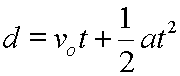## StatsMiniBlog: Significance tests. Step one.Moving through from  estimating how the mean of a sample might reflect the mean of the population at large, we want to see if there are differences between two (or more) groups. This is usually done by ‘doing a statistical test’. What we won’t be dealing with here is the maths that derives these, the processes of creating the test score and checking this through to an answer, but the core concept. What is it we’re doing when we ‘do a statistical test’

We’re asking ‘what’s the likelihood that the results I’ve got from these two groups are different only because of the play of chance?’

Then the p-value gives us an answer.

p=0.01; the likelihood of the results from these two groups being found purely because of chance is 1 in 100.

Exactly what we are looking at when we examine “the results I’ve got from these two groups”, and so which statistical test to use, is where we’re going with the next post.

– Archi

(Visited 166 times, 1 visits today)